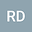CONVOLUTION EQUATIONS ON THE ABELIAN GROUP $\cA(-1,1)$
•The interval $j=[-1,1]$ turns into an Abelian group $\cA(\cJ)$ under the group operation $x+_\cJ y:=(x+y)(1+xy)^{-1},\qquad x,y\in\cJ$. This enables definition of the invariant measure $d_\cJ x=(1-x^2)^{-1}dx$ and the Fourier transform $\cF_\cJ$ on the interval $\cJ$ and, as a consequence, we can consider Fourier convolution operators $W^0_{\cJ,\cA}:=\cF_\cJ^{-1}\cA\cF_\cJ$ on $\cJ$. This class of convolutions includes celebrated Prandtl, Tricomi and Lavrentjev-Bitsadze equations and, also, differential equations of arbitrary order with the natural weighted derivative $\fD_\cJ u(x)=-(1-x^2)u’(x)$, $t\in\cJ$. Equations are solved in the scale of Bessel potential $\bH^s_p(\cJ,d_\cJ x)$, $1\leqslant p\leqslant\infty$, and H\”older-Zygmound $\bZ^\nu(\cJ,(1-x^2)^\mu)$, $0<\mu,\nu<\infty$ spaces, adapted to the group $\cA(\cJ)$. Boundedness of convolution operators (the problem of multipliers) is discussed. The symbol $\cA(\xi)$, $\xi\in\bR$, of a convolution equation $W^0_{\cJ,\cA}u=f$ defines solvability: the equation is uniquely solvable if and only if the symbol $\cA$ is elliptic. The solution is written explicitely with the help of the inverse symbol. We touch shortly the multidimensional analogue-the Abelian group $\cA(\cJ^n)$.